Study Materials: ncert solutions

Our ncert solutions for Miscellaneous Exercise on Chapter - 9 (Available) Class 11 maths 9. Sequences and Series - ncert solutions - Toppers Study is the best material for English Medium students cbse board and other state boards students.

Solutions ⇒ Class 11th ⇒ Mathematics ⇒ 9. Sequences and Series

# Miscellaneous Exercise on Chapter - 9 (Available) Class 11 maths 9. Sequences and Series - ncert solutions - Toppers Study

Topper Study classes prepares ncert solutions on practical base problems and comes out with the best result that helps the students and teachers as well as tutors and so many ecademic coaching classes that they need in practical life. Our ncert solutions for Miscellaneous Exercise on Chapter - 9 (Available) Class 11 maths 9. Sequences and Series - ncert solutions - Toppers Study is the best material for English Medium students cbse board and other state boards students.

## Miscellaneous Exercise on Chapter - 9 (Available) Class 11 maths 9. Sequences and Series - ncert solutions - Toppers Study

CBSE board students who preparing for class 11 ncert solutions maths and Mathematics solved exercise chapter 9. Sequences and Series available and this helps in upcoming exams 2023-2024.

### You can Find Mathematics solution Class 11 Chapter 9. Sequences and Series

• All Chapter review quick revision notes for chapter 9. Sequences and Series Class 11
• NCERT Solutions And Textual questions Answers Class 11 Mathematics
• Extra NCERT Book questions Answers Class 11 Mathematics
• Importatnt key points with additional Assignment and questions bank solved.

NCERT Solutions do not only help you to cover your syllabus but also will give to textual support in exams 2023-2024 to complete exercise -9 maths class 11 chapter 9 in english medium. So revise and practice these all cbse study materials like class 11 maths chapter -9 in english ncert book. Also ensure to repractice all syllabus within time or before board exams for ncert class 11 maths ex -9 in english.

See all solutions for class 11 maths chapter 9 exercise 9 in english medium solved questions with answers.

### Miscellaneous Exercise on Chapter - 9 (Available) class 11 Mathematics Chapter 9. Sequences and Series

• Miscellaneous Exercise On Chapter - 9 (Available) Class 11 Maths 9. Sequences And Series - Ncert Solutions - Toppers Study
• Class 11 Ncert Solutions
• Solution Chapter 9. Sequences And Series Class 11
• Solutions Class 11
• Chapter 9. Sequences And Series Miscellaneous Exercise On Chapter - 9 (Available) Class 11

## Miscellaneous Exercise on Chapter - 9 (Available) Class 11 maths 9. Sequences and Series - ncert solutions - Toppers Study

Miscellaneous Exercise on Chapter - 9

Q1. Show that the sum of (m + n)th and (m – n)th terms of an A.P. is equal to twice the mth term.
Solution:
Let a and d be the first term and the common difference of the A.P. respectively. It is known that the kth term of an A. P. is given by
ak = a + (k –1) d
∴ am + n = a + (m + n –1) d
am – n = a + (m – n –1) d
am = a + (m –1) d
∴ am + n + am – n = a + (m + n –1) d + a + (m – n –1) d
= 2a + (m + n –1 + m – n –1) d
= 2a + (2m – 2) d
= 2a + 2 (m – 1) d
=2 [a + (m – 1) d]
= 2am
Thus, the sum of (m + n)th and (m – n)th terms of an A.P. is equal to twice the mth term.

Q2. If the sum of three numbers in A.P., is 24 and their product is 440, find the numbers.
Solution:
Let the three numbers in A.P. be a – d, a, and a + d.
According to the given information,
(a – d) + (a) + (a + d) = 24 … (1)
⇒ 3a = 24
∴ a = 8
(a – d) a (a + d) = 440 … (2)
⇒ (8 – d) (8) (8 + d) = 440
⇒ (8 – d) (8 + d) = 55
⇒ 64 – d2 = 55
⇒ d2 = 64 – 55 = 9
⇒ d = ± 3
Therefore, when d = 3, the numbers are 5, 8, and 11 and when d = –3, the numbers are 11, 8, and 5.
Thus, the three numbers are 5, 8, and 11.

Q3. Let the sum of n, 2n, 3n terms of an A.P. be S1, S2 and S3, respectively, show that S3 =3 (S2– S1).
Solution:
Let a and b be the first term and the common difference of the A.P. respectively. Therefore,Q4. Find the sum of all numbers between 200 and 400 which are divisible by 7.
Solution:
The numbers lying between 200 and 400, which are divisible by 7, are
203, 210, 217 … 399
∴ First term, a = 203
Last term, l = 399
Common difference, d = 7

Let the number of terms of the A.P. be n.
∴ an = 399 = a + (n –1) d
⇒ 399 = 203 + (n –1) 7
⇒ 7 (n –1) = 196
⇒ n –1 = 28
⇒ n = 29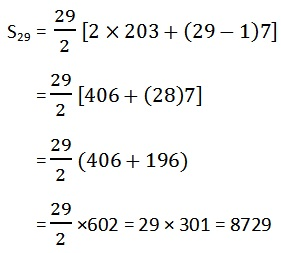Sum of 29 terms is 8729.

Q5. Find the sum of integers from 1 to 100 that are divisible by 2 or 5.

Solution:

Integers from 1 to 100 which are divisible by 2 are 2, 4, 6, 8 ................. 100.

which form A.P : 2, 4, 6, 8 ................. 100.

Case-I

a = 2, d = d2 - d1 ⇒ 4 - 2 = 2 , an = 100,

an = a + (n - 1)d​

100 = 2 + (n - 1)2

100 - 2 = (n - 1)2

98 = (n - 1)2

n - 1 = 98/2

n - 1 = 49

n = 49 + 1

n = 50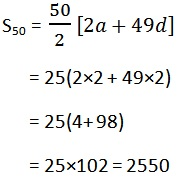Case-II

The integers between 1 to 100 divisible by 5.

5, 10, 15 ..................... 100.

a = 5, d = 10 - 5 = 5,

an = 100

an = a + (n - 1)d​

⇒100 = 5 + (n - 1)5

⇒100 - 5 =  (n - 1)5

⇒95/5 = n - 1

⇒19 = n - 1

⇒n = 19 + 1

⇒n = 20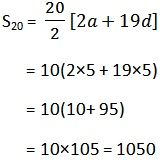Q6. Find the sum of all two digit numbers which when divided by 4, yields 1 as remainder.

Solution:

The two-digit numbers, which when divided by 4, yield 1 as remainder, are as

A.P: 13, 17, … 97.
This series forms an A.P.

a = 13, d = 17-13 = 4 an = 97

an = a + (n –1) d
∴ 97 = 13 + (n –1) (4)
⇒ 4 (n –1) = 84
⇒ n – 1 = 21
⇒ n = 22
Sum of n terms of an A.P. is given by,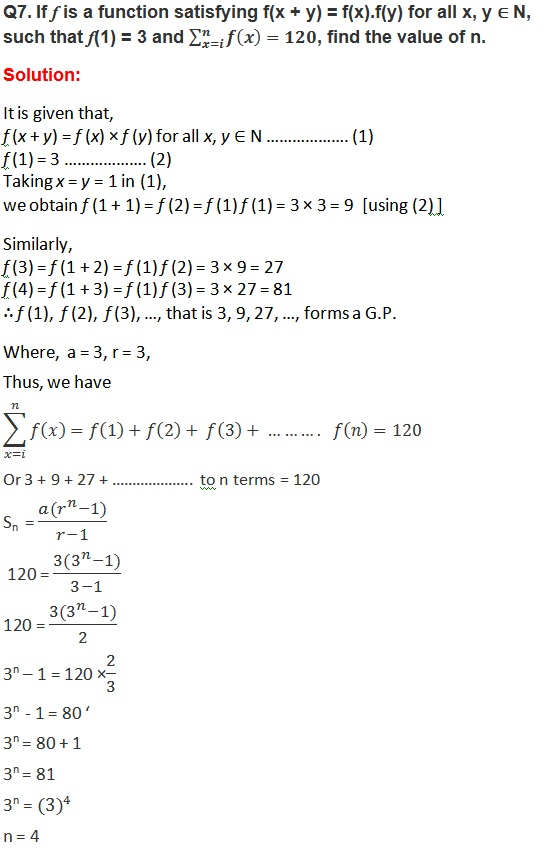Q8. The sum of some terms of G.P. is 315 whose first term and the common ratio are 5 and 2, respectively. Find the last term and the number of terms.

Solution:

It is given that a = 5, r = 2, Sn = 315

n = ? and an = ?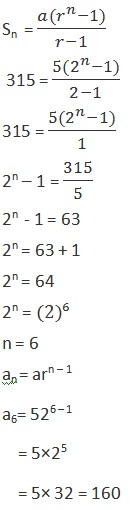Q9. The first term of a G.P. is 1. The sum of the third term and fifth term is 90. Find the common ratio of G.P.

Solution:

Let a and r be the first term and the common ratio of the G.P, respectively.

T1 = a = 1

T2 = ar = 1×r = r

T3 = ar2 = 1× r= r2

T4 = ar3 = 1× r= r3

T5 = ar4 = 1× r= r4

Given: T3 + T5 = 90

r2 +  r4 = 90

⇒  r+ r2 - 90 =0

Let  r2 = y then gives;

y2 + y - 90 = 0

y2 + 10y - 9y - 90 = 0

y(y + 10) -9(y + 10) = 0

(y + 10)(y - 9) = 0

y + 10 = 0, y - 9 = 0

y = -10 (Not to be taken) , y = 9

y = 9 ⇒ r2 = 9 ⇒ r = √9 ⇒ r = ± 3

Therefore, common ratios are 3, - 3.

Q10. The sum of three numbers in G.P. is 56. If we subtract 1, 7, 21 from these numbers in that order, we obtain an arithmetic progression. Find the numbers.

Solution:

Let the three numbers in G.P. be a, ar, and ar2.
According to condition,
a + ar + ar2 = 56   ……………….… (1)

Now, a – 1, ar – 7, ar2 – 21 are in A.P

therefore,

a1 = a – 1,

a2 = ar – 7,

a3 = ar2 – 21

a2 - a1 = a3 - a​2
∴ (ar – 7) – (a – 1) = (ar2 – 21) – (ar – 7)
⇒ ar – a – 6 = ar2 – ar – 14
⇒ar2 – 2ar + a = 8
⇒a – 2ar + ar2   = 8  ........................ (2)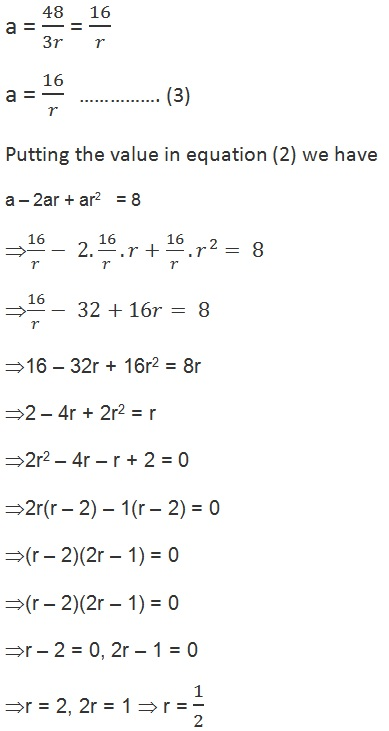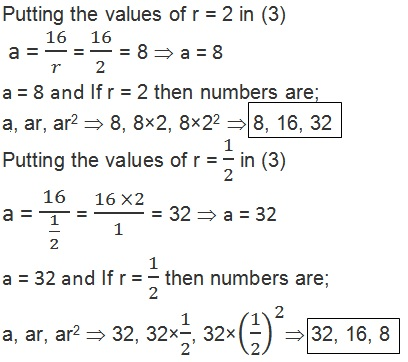Q11. A G.P. consists of an even number of terms. If the sum of all the terms is 5 times the sum of terms occupying odd places, then find its common ratio.

Solution:

Let the G.P be T1, T2, T3, T4 ................. T2n

It is given that there are even numbers of terms.

numbers of terms = 2n

S = T1 + T2 + T3 + T4 + .................+ T2n

S = a + ar + ar2 + ……………….

First term = a, common ratio = r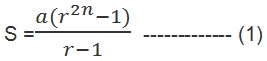Let be sum of terms occupying odd places

S1 = T1 + T3 + T5 + .................+ T2n-1

S1 = a + ar2 + ar4 + ……………….

First term = a, common ratio = r2

Total terms in this series = n  (half of 2n)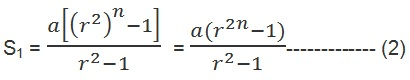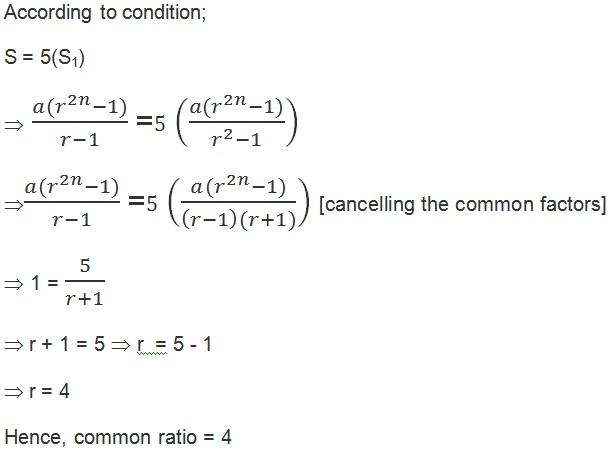Q12. The sum of the first four terms of an A.P. is 56. The sum of the last four terms is 112. If its first term is 11, then find the number of terms.

Solution:

S4 = 56, a = 11, Let total terms in A.P be n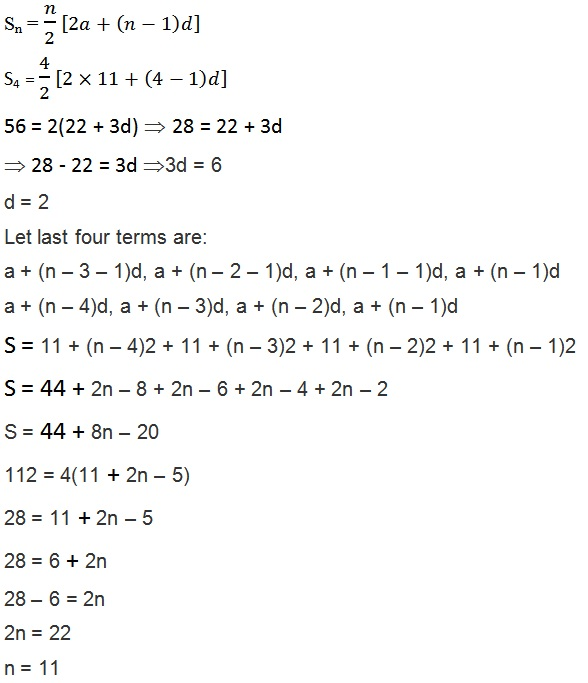Important Study materials for classes 06, 07, 08,09,10, 11 and 12. Like CBSE Notes, Notes for Science, Notes for maths, Notes for Social Science, Notes for Accountancy, Notes for Economics, Notes for political Science, Noes for History, Notes For Bussiness Study, Physical Educations, Sample Papers, Test Papers, Mock Test Papers, Support Materials and Books.Mathematics Class - 11th

NCERT Maths book for CBSE Students.

books

## Study Materials List:

##### Solutions ⇒ Class 11th ⇒ Mathematics
1. Sets
2. Relations and Functions
3. Trigonometric Functions
4. Principle Of Mathematical Induction
5. Complex Numbers and Quadratic Equations
6. Linear Inequalities
7. Permutations and Combinations
8. Binomial Theorem
9. Sequences and Series
10. Straight Lines
11. Conic Sections
12. Introduction to Three Dimensional Geometry
13. Limits and Derivatives
14. Mathematical Reasoning
15. Statistics
16. Probability

New Books